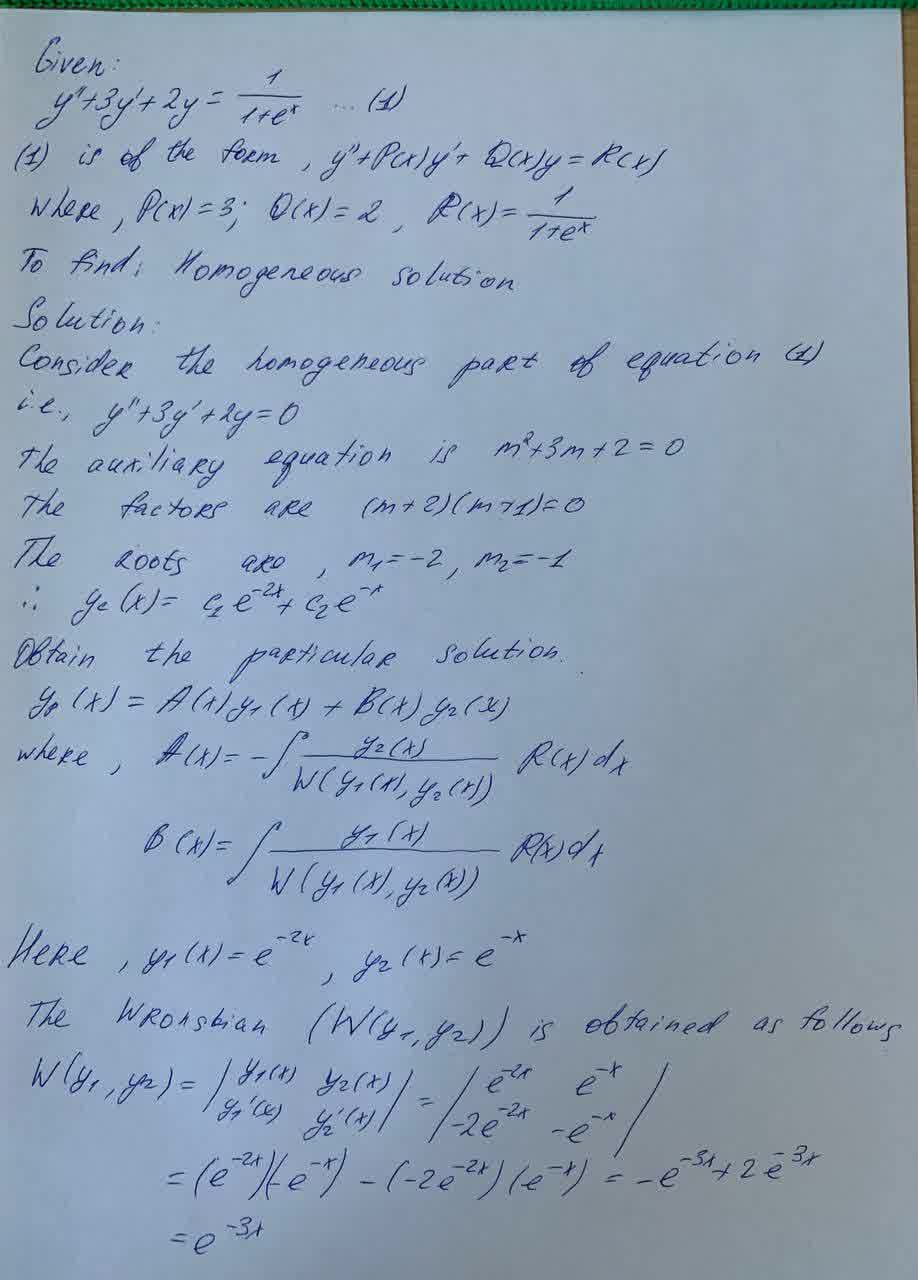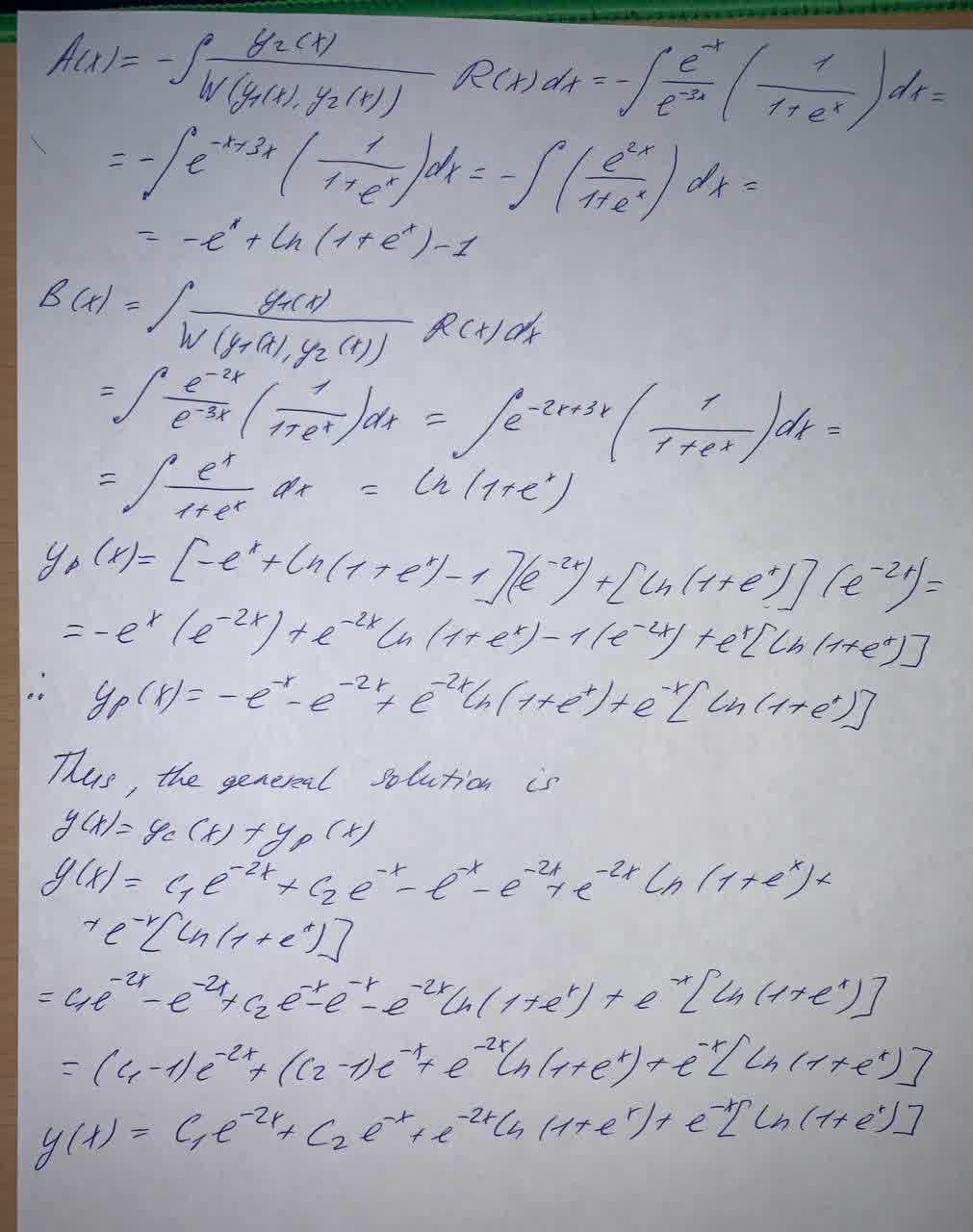Question# Solve the differential equation by variation of parameters. y" + 3y' +2y = \frac{1}{1+e^x}

Differential equations
ANSWEREDSolve the differential equation by variation of parameters
$$\displaystyle{y}\text{}+{3}{y}'+{2}{y}={\frac{{{1}}}{{{1}+{e}^{{x}}}}}$$